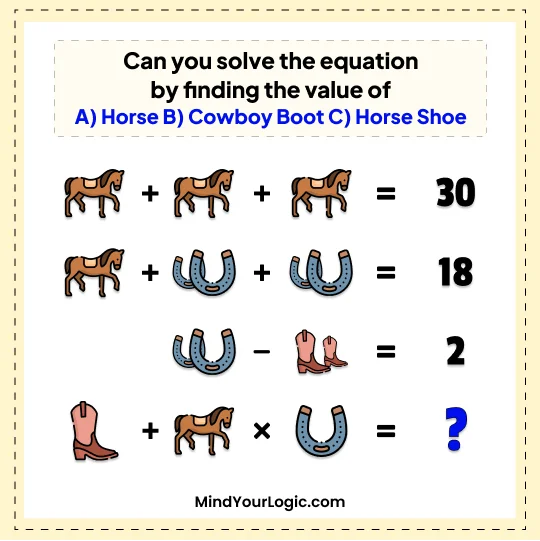# Horse Cowboy Boot and Horseshoe Viral - Math Riddle

###### 125.Math Riddles
```Can you solve the equation by finding the value of
A) Horse
B) Cowboy Boot
C) Horse Shoe```• `Horse = 10, Cowboy Boot = 1 ,Horse Shoe= 2`
```Explanation :

Horse = 10, Cowboy Boot = 1 ,Horse Shoe= 2

= Step 1 =
3 Horse = 30
= Horse = 10 .....(A)

= Step 2 =
1 Horse + 2 Horse Shoe + 2 Horse Shoe = 18
10 + 4 Horse Shoe =18
HorseShoe = 2 .....(C)

= Step 3 =
2 Horse Shoe - 2 Cowboy Boot = 2
4 - 2 Cowboy Boot = 2
= Cowboy Boot = 1 ... (B)

= Step4 =
Cowboy Boot + Horse * Horse Shoe = 1+10*2 = 21 ```